Publicité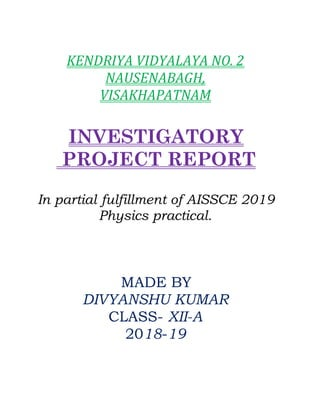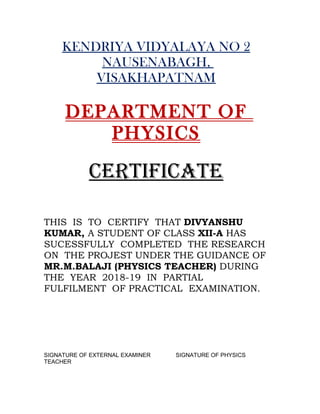Publicité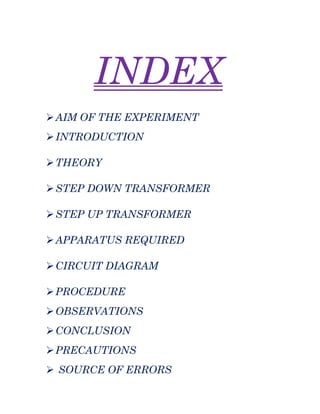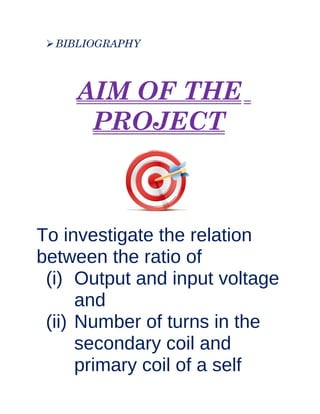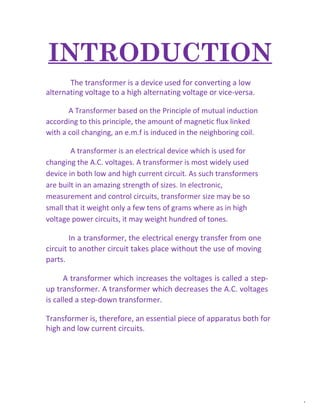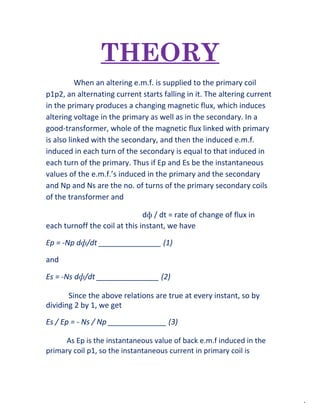Publicité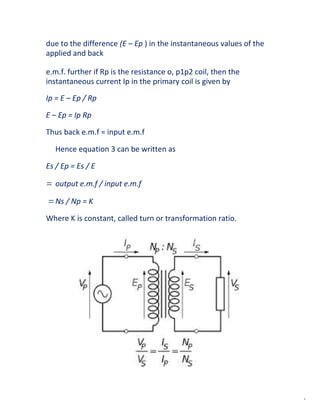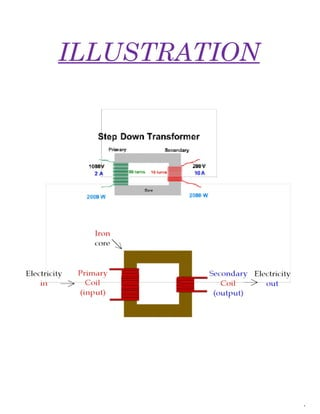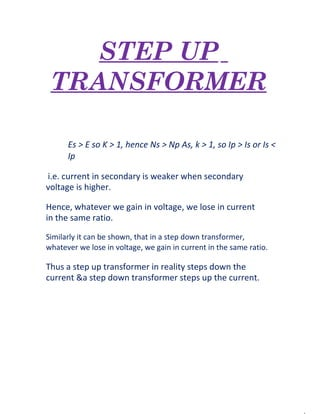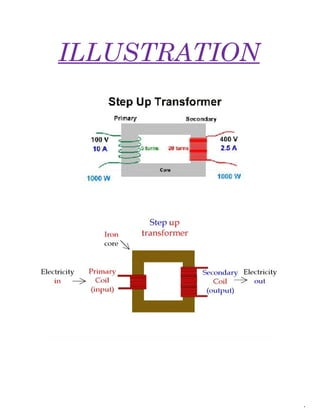Publicité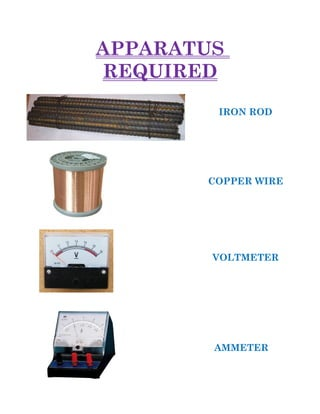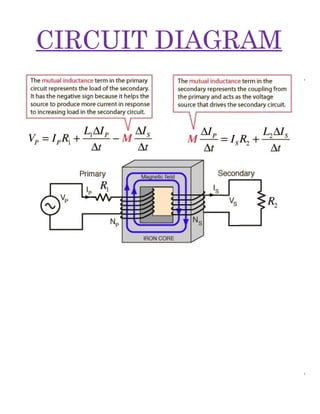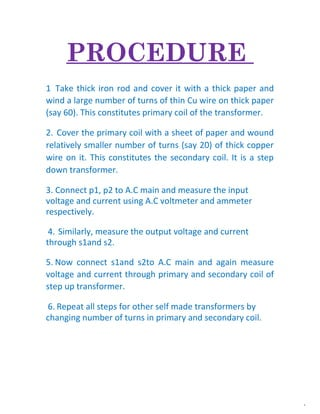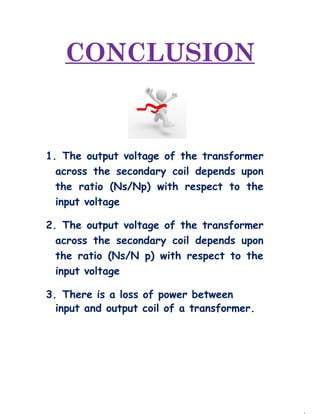Publicité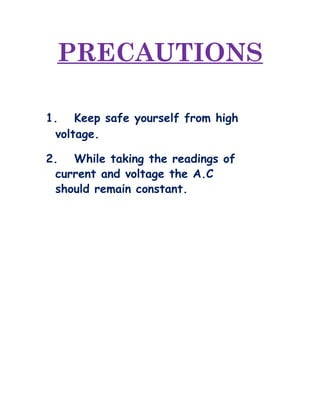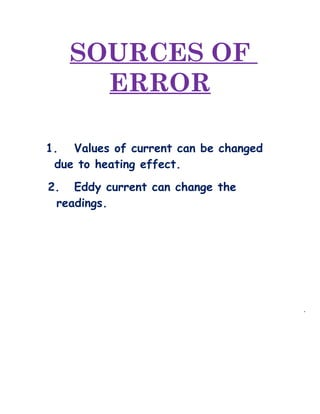Prochain SlideSharePhysics Investigatory Project Class 12
Chargement dans ... 3
1 sur 22
Publicité

### Physics Investigatory Project

1. M.B.S PUBLIC SCHOOL KENDRIYA VIDYALAYA NO. 2 NAUSENABAGH, VISAKHAPATNAM INVESTIGATORY PROJECT REPORT In partial fulfillment of AISSCE 2019 Physics practical. MADE BY DIVYANSHU KUMAR CLASS- XII-A 2018-19
2. KENDRIYA VIDYALAYA NO 2 NAUSENABAGH, VISAKHAPATNAM DEPARTMENT OF PHYSICS CERTIFICATE THIS IS TO CERTIFY THAT DIVYANSHU KUMAR, A STUDENT OF CLASS XII-A HAS SUCESSFULLY COMPLETED THE RESEARCH ON THE PROJEST UNDER THE GUIDANCE OF MR.M.BALAJI (PHYSICS TEACHER) DURING THE YEAR 2018-19 IN PARTIAL FULFILMENT OF PRACTICAL EXAMINATION. SIGNATURE OF EXTERNAL EXAMINER SIGNATURE OF PHYSICS TEACHER
3. ACKNOWLEDGEMEN T I would like to express my special thanks of gratitude to my Physics Teacher Mr.M.BALAJI as well as our School Principal Mr.Nishikant Aggarwal who gave the golden opportunity to do this wonderful project which also helped me in doing a lot of research work and I came to know about so many new things. I am really thankful to them.
4. INDEX AIM OF THE EXPERIMENT INTRODUCTION THEORY STEP DOWN TRANSFORMER STEP UP TRANSFORMER APPARATUS REQUIRED CIRCUIT DIAGRAM PROCEDURE OBSERVATIONS CONCLUSION PRECAUTIONS  SOURCE OF ERRORS
5. BIBLIOGRAPHY AIM OF THE PROJECT To investigate the relation between the ratio of (i) Output and input voltage and (ii) Number of turns in the secondary coil and primary coil of a self
6. designed transformer.
7. INTRODUCTION The transformer is a device used for converting a low alternating voltage to a high alternating voltage or vice-versa. A Transformer based on the Principle of mutual induction according to this principle, the amount of magnetic flux linked with a coil changing, an e.m.f is induced in the neighboring coil. A transformer is an electrical device which is used for changing the A.C. voltages. A transformer is most widely used device in both low and high current circuit. As such transformers are built in an amazing strength of sizes. In electronic, measurement and control circuits, transformer size may be so small that it weight only a few tens of grams where as in high voltage power circuits, it may weight hundred of tones. In a transformer, the electrical energy transfer from one circuit to another circuit takes place without the use of moving parts. A transformer which increases the voltages is called a step- up transformer. A transformer which decreases the A.C. voltages is called a step-down transformer. Transformer is, therefore, an essential piece of apparatus both for high and low current circuits.
8. THEORY When an altering e.m.f. is supplied to the primary coil p1p2, an alternating current starts falling in it. The altering current in the primary produces a changing magnetic flux, which induces altering voltage in the primary as well as in the secondary. In a good-transformer, whole of the magnetic flux linked with primary is also linked with the secondary, and then the induced e.m.f. induced in each turn of the secondary is equal to that induced in each turn of the primary. Thus if Ep and Es be the instantaneous values of the e.m.f.’s induced in the primary and the secondary and Np and Ns are the no. of turns of the primary secondary coils of the transformer and dф / dt = rate of change of flux in each turnoff the coil at this instant, we have Ep = -Np dф/dt _______________ (1) and Es = -Ns dф/dt _______________ (2) Since the above relations are true at every instant, so by dividing 2 by 1, we get Es / Ep = - Ns / Np ______________ (3) As Ep is the instantaneous value of back e.m.f induced in the primary coil p1, so the instantaneous current in primary coil is
9. due to the difference (E – Ep ) in the instantaneous values of the applied and back e.m.f. further if Rp is the resistance o, p1p2 coil, then the instantaneous current Ip in the primary coil is given by Ip = E – Ep / Rp E – Ep = Ip Rp Thus back e.m.f = input e.m.f Hence equation 3 can be written as Es / Ep = Es / E = output e.m.f / input e.m.f =Ns / Np = K Where K is constant, called turn or transformation ratio.
10. STEP DOWN TRANSFORMER Es < E so K < 1, hence Ns < Np If Ip = value of primary current at the same instant And Is = value of secondary current at this instant, then Input power at the instant = Ep Ip And Output power at the same instant = Es Is If there are no losses of power in the transformer, then Input power = output power Or Ep Ip = Es Is Or Es / Ep = Ip / Is = K
11. ILLUSTRATION
12. STEP UP TRANSFORMER Es > E so K > 1, hence Ns > Np As, k > 1, so Ip > Is or Is < Ip i.e. current in secondary is weaker when secondary voltage is higher. Hence, whatever we gain in voltage, we lose in current in the same ratio. Similarly it can be shown, that in a step down transformer, whatever we lose in voltage, we gain in current in the same ratio. Thus a step up transformer in reality steps down the current &a step down transformer steps up the current.
13. ILLUSTRATION
14. APPARATUS REQUIRED IRON ROD COPPER WIRE AMMETER VOLTMETER
15. CIRCUIT DIAGRAM
16. PROCEDURE 1 Take thick iron rod and cover it with a thick paper and wind a large number of turns of thin Cu wire on thick paper (say 60). This constitutes primary coil of the transformer. 2. Cover the primary coil with a sheet of paper and wound relatively smaller number of turns (say 20) of thick copper wire on it. This constitutes the secondary coil. It is a step down transformer. 3. Connect p1, p2 to A.C main and measure the input voltage and current using A.C voltmeter and ammeter respectively. 4. Similarly, measure the output voltage and current through s1and s2. 5. Now connect s1and s2to A.C main and again measure voltage and current through primary and secondary coil of step up transformer. 6. Repeat all steps for other self made transformers by changing number of turns in primary and secondary coil.
17. OBSERVATION TABLE S.No. No. of turns in primary coil (Np) No. of turns in secondary coil (Ns) Voltage in primary coil (Ep) Curren t in P. coil Ip Voltage in secondary coil (Es) Current in secondary coil Is Loss of power B 1 . 2 . 3 . 4 . 5 . 6 . 7 . 8 .
18. CONCLUSION 1. The output voltage of the transformer across the secondary coil depends upon the ratio (Ns/Np) with respect to the input voltage 2. The output voltage of the transformer across the secondary coil depends upon the ratio (Ns/N p) with respect to the input voltage 3. There is a loss of power between input and output coil of a transformer.
19. PRECAUTIONS 1. Keep safe yourself from high voltage. 2. While taking the readings of current and voltage the A.C should remain constant.
20. SOURCES OF ERROR 1. Values of current can be changed due to heating effect. 2. Eddy current can change the readings.
21. BIBLIOGRAPHY HELP FROM INTERNET INFORMATION FROM LAB MANUAL TEACHER’S GUIDANCE
Publicité